Join the forum, it's quick and easy

Would you like to react to this message? Create an account in a few clicks or log in to continue.

# [討論]請問各位先進都怎麼進行偵錯呢?

3 posters##[討論]請問各位先進都怎麼進行偵錯呢?

小弟為LISP的新手，書本買了2.3本，也自己動手寫了一些簡單的LISP，不過對於偵錯的用法以及"眉角"實在是非常的生疏，曾經爬文看過一些偵錯的教學，但是不知道是不是因為沒有學過相關的程式語言，所以似乎邏輯無法暢通，

(一)主程式內容

(defun c:ff ()(c:ling)(c:ffra)(c:ffr)(c:lins)(c:ffrb)
(defun c:ffra ()
(if (< DDDD 0)
(exit)
)
(if (not (new_dialog "ffr" DDDD))
(exit)
)
(action_tile "cancel" "(exit)")
(action_tile "accept" "(ffrb)(done_dialog4)")
(start_dialog)
(done_dialog)
)
(defun c:ffrb ()
(setq a (get_tile "icon1"))
(setq d (atof (get_tile "icon3")))
(setq h (atof (get_tile "icon4")))
(setq dhtext (strcat "備註:等高線首曲線間距h＝"
(rtos h 2 0)
"公尺,方格(丘塊)邊長="
(rtos d 2 0)
"公尺"
)
)
(setq e (get_tile "icon5"))
(if (= a "1")
(setq sss 100.0)
(setq sss 1.0)
)
(if (= e "1")
(setq ha 1)
(setq ha 2)
)
(if (= a "1")
(setq d (* d sss)
h (* h sss)
)
)
)

(defun c:ffr ()(wp)
(setq p1 (getpoint (if1 "\nEnter 1st corner:" "\n輸入第一角點:")))
(setq p2 (getcorner p1 (if1 "\nEnter 2nd corner:" "\n輸入第二角點:")))
(setq sh (cdr (assoc 8 (entget (car (entsel (IF1 "\nSelect pline:" "\n選取等高線:")))))))
(setq pq1 (getpoint (IF1 "\nEnter location of text:" "\n輸入檢討表位置:")))
(setq no 0 areatext 0 suareax 0 agareax 0 areaqx 0)
(setq bbp (getvar "blipmode"))
(setvar "blipmode" 0)
(setq x (abs (- (car p2) (car p1)))
)
(setq p3a (list (car p2) (cadr p1)))
(setq p3b (list (car p1) (cadr p2)))
(setq ax (angle p1 p3a)
bx (angle p1 p3b)
)
(setq xn (+ 1 (fix (/ x d))))
(setq yn (+ 1 (fix (/ x d))))
(setq pp  p1
pp1 p1
p3a (polar p1 ax (* xn d))
p3b (polar p1 bx (* yn d))
)
(repeat yn
(repeat xn
(setq p2 (polar p1 ax d)
p3 (polar p2 bx d)
p4 (polar p1 bx d)
)
(command "layer" "m" "00" "")
(command "_pline" p1 p2 p3 p4 "c")
(setq el (ssget "1"))
(setq s (ssget "cp" 1  (list(cons 0 "LWPOLYLINE,polyline,arc,line,spline")(cons 8 "00"))))
(if (/ = s nil)
(progn
(setq snj 0)
(command "zoom" "w" p1 p3)
(setq p1p2s (ssget "c" p1 p2 (list (cons 8 sh))))
(if (/ = p1p2s nil) (setq snj (+ (sslength p1p2s) snj)))
(setq p1p2s (ssget "c" p2 p3 (list (cons 8 sh))))
(if (/ = p1p2s nil)(setq snj (+ (sslength p1p2s) snj)))
(setq p1p2s (ssget "c" p3 p4 (list (cons 8 sh))))
(if (/ = p1p2s nil)(setq snj (+ (sslength p1p2s) snj)))
(setq p1p2s (ssget "c" p4 p1 (list (cons 8 sh))))
(if (/ = p1p2s nil) (setq snj (+ (sslength p1p2s) snj)))
(command "zoom" "p")
(command "copy" sa "" "@" "@")
(command "region" "1" "")
(setq sal (ssget "1"))
(command "region" el "")
(setq ela (ssget "1"))
(command "intersect" sal ela "")
(setq sall (ssget "1"))
(command "area" "e" sall)
(if (= sss 100.0)
(setq areaq (/ (getvar "area") 10000.0))
(setq areaq (getvar "area"))
)
(hatchset)
(setq suarea (* su 100.0 areaq))
(setq agarea (* (atof ag) areaq))
(setq areaqx (+ areaqx areaq))
(setq suareax (+ suareax suarea))
(setq agareax (+ agareax agarea))
(textw)(textset)
(command "erase" sal ""))
(command "erase" el ""))
(setq p1 (polar p1 ax d))
)
(setq pp (polar pp bx d)p1 pp)
)
(boxline)
(linex）
(seq suxx (/ suareax areaqx) agxx (/ agareax areaqx))
(command "_style" "china" "ch,chineset" "" "" "" "" "" "N")
(setq pq0 (polar pq1 (* 0.5 pi) (* 0.3 s6)))
(setq pq2 (polar pq1 0 s6))
(setq pq3 (polar pq2 0 s6))
(setq pq4 (polar pq3 0 s6))
(setq pq5 (polar pq4 0 s6))
(setq pq6 (polar pq5 0 s6))
(setq pq7 (polar pq6 0 s6))
(setq qq1 (polar qq1 (* 1.5 pi) (* 0.7 s6)))
(setq qq2 (polar qq1 (* 1.5 pi) s4))
(setq qq3 (polar qq2 (* 1.5 pi) s4))
(command "text" qq1 (* sss 2.0) 0 (strcat "總平均坡度= " (rtos suareax 2 2) "/" (rtos areaqx 2 2) "=" (rtos suxx 2 2) "%"))
(command "text" qq2 (* sss 2.0) 0 (strcat "總平均角度= " (rtos agareax 2 2) "/" (rtos areaqx 2 2) "=" (rtos agxx 2 2) "%%D"))
(command "text" qq3 (* sss 2.0) 0 dhtext)
(command "text" pq0 (* sss 3.0) 0 "建築技術規則第十三章平均坡度計算表:")(c:cr 7)
(command "text" pq1 (* sss 2.0) 0 "編號(no)")(c:cr 7)
(command "text" pq2 (* sss 2.0) 0 "坡度(％)")(c:cr 7)
(command "text" pq3 (* sss 2.0) 0 "坡角(％％d)")(c:cr 7)
(command "text" pq4 (* sss 2.0) 0 "交點數(N)")(c:cr 7)
(command "text" pq5 (* sss 2.0) 0 "面積(m^2)")(c:cr 7)
(command "text" pq6 (* sss 2.0) 0 "坡度比")(c:cr 7)
(command "text" pq7 (* sss 2.0) 0 "角度比")(c:cr 7)
(setq qq5 (polar qq5 (* 1.5 pi) s5))
(setq qq6 (polar qq6 (* 1.5 pi) s5))
(setq qq7 (polar qq7 (* 1.5 pi) s5))
(command "text" qq5 (* sss 2.0) 0 (rtos areaqx 2 2))(c:cr 7)
(command "text" qq6 (* sss 2.0) 0 (rtos areaqx 2 2))(c:cr 7)
(command "text" qq7 (* sss 2.0) 0 (rtos areaqx 2 2))(c:cr 7)
(setvar "textstyle" texty)
(setvar "blipmode" bbp)
(princ)
)
(defun c:wp ()
(prompt (if1 "\nSelect pline:" "\n選取地界線:"))
(c:listid)
(setq sa s 1 listxy)
)
(defun c:cr (n) (command "change" "l" "" "p" "c" n ""))

(defun c:listid (/ se sn ename ee c10 x y z xy xy1 j ett et e0)
(setq s (entsel) et (car s))
(setq se (entget (car s)) j 0 listp '() listxy '())
(setq sn (length se))
(setq ename (strcase (cdr (assoc 0 se))))
(if (= ename "LWPOLYLINE")
(repeat sn
(progn
(setq ee (nth j se))
(setq c10 (car ee))
(if (= 10 c10)
(progn
(setq xy (list (strcat (rtos x 2 2) "," (rtos y 2 2))))
(setq xy1 (list (list x y)))
(setq listp (append listp xy))
(setq listxy (append listxy xy1))
)
)
)
(setq j (+ j 1))
)
)
(if (= ename "POLYLINE")
(progn
(setq ett (entnext et))
(while (/ = ett nil)
(setq et (entget ett))
(setq e0 (cdr (assoc 0 et)))
(if (= e0 "VERTEX")
(progn
(setq ee (cdr (assoc 10 et)))
(setq xy (list (strcat (rtos x 2 2) "," (rtos y 2 2) "," (rtos z 2 2))))
(setq xy1 (list (list x y z)))
(setq listp (append listp xy))
(setq listxy (append listxy xy1))
)
)
(setq ett (entnext ett))
)
)
)
(setq listn (length listp))

(print listp)
(print listxy)
)
(defun c:ling ()
(setq c1 (getvar "clayer") stext (getvar "textstyle")))

(defun c:lins ()
(setvar "clayer" c1)
(setvar "textstyle" stext)
)
)
)
)
)

(二)DCL對話框程式

ffr:dialog{
label = "第十三章之平均坡度分析圖表";
key = "label";
initial_focus  = "cancel";
:column {
:boxed_row{
label="圖面單位";
}}
:boxed_column{
:row{:edit_box{key="icon3";label= "方格尺寸=";value=25;}:text{label= "公尺";}}
:row{:edit_box{key="icon4";label= "單位落差=";value=5;}:text{label= "公尺"; }}
}
:boxed_row{label= "繪圖時之色彩";
}
}
ok_cancel;
}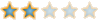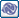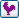##回復： [討論]請問各位先進都怎麼進行偵錯呢?

1.計算每個方格邊長上與等高線交點數目，總計每個方格4邊的交點數總和，代入坡度計算公式計算該坵塊的代表坡度。
2.將被坵塊方格分割的計畫範圍，分別依每個方格的代表坡度加權計算整個計畫區平均坡度。
3.輸出坡度分析計算的表格。

P.S這個LISP可能在實務使用上比較冷門，但因小弟正式從事這類型工作，LISP真的可大大提升效率，希望各位大大給予指導~感謝感謝!!!##回復： [討論]請問各位先進都怎麼進行偵錯呢?VLISP 就會自動找到相對應的左括號. 並反藍...!! 我都是這樣檢查..

1. IF1 副程式.程式內找不到.
2.  1 為數字.  不得當變數名.
(setq sa s 1 listxy)  就是將 listxy 設給  1  這樣不對.請再checkDCL  對齊後註記 大括弧.位置.LISP下載
https://db.tt/wCakYzV3
DCL下載
https://db.tt/JMNh5zMQ

Tiger&蘋果爸 寫到:謝謝熱心圖文回覆~< 積分 +1>
[公告]關於團隊成員的回文及貢獻shenhung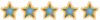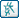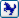##回復： [討論]請問各位先進都怎麼進行偵錯呢?

ssget應該是沒有"1"的選擇，可能是建檔時輸入錯誤，Atsai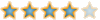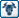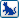##回復： [討論]請問各位先進都怎麼進行偵錯呢?

1.非常感謝S大大的指導，原來可以用這方式檢查括號，實在獲益良多，可否在請教一下S大大，是否有地方可以查詢或說明相關函數及指令的用法或範例呢??因為其中部分函數指令，小弟並不熟悉，著實增加偵錯的難度啊!!!另外，有關副程式，直接寫在一起或分開編輯載入後再呼叫，是否會有差異??

2.A大大您好，您說的沒錯，這是水保裡面的坡度分析，不過小弟我的功力並未到家，自己沒辦法寫這麼龐大的LISP，所以是爬了文章在網路上搜尋到一篇論文，以紙本建檔的，且因為論文的字形部分數字1與英文小寫l，實在難以辨識，再加上部分函數指令並不熟悉，可能真的建檔時輸入了很多錯誤，若真有需要，小弟可以提供在網路上搜尋到的PDF檔，再請A大大指導一下。##回復： [討論]請問各位先進都怎麼進行偵錯呢?Atsai##回復： [討論]請問各位先進都怎麼進行偵錯呢?shenhung##回復： [討論]請問各位先進都怎麼進行偵錯呢?##回復： [討論]請問各位先進都怎麼進行偵錯呢?Atsai##回復： [討論]請問各位先進都怎麼進行偵錯呢?

A大您好~##回復： [討論]請問各位先進都怎麼進行偵錯呢?Atsai##回復： [討論]請問各位先進都怎麼進行偵錯呢?##回復： [討論]請問各位先進都怎麼進行偵錯呢?

Atsai 在 2022-06-24, 16:47 作了第 1 次修改Atsai##回復： [討論]請問各位先進都怎麼進行偵錯呢?